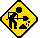## Texas Instruments TI-85

Datasheet legend
Ab/c: Fractions calculation
AC: Alternating current
BaseN: Number base calculations
Card: Magnetic card storage
Cmem: Continuous memory
Cond: Conditional execution
Const: Scientific constants
Cplx: Complex number arithmetic
DC: Direct current
Eqlib: Equation library
Exp: Exponential/logarithmic functions
Fin: Financial functions
Grph: Graphing capability
Hyp: Hyperbolic functions
Intg: Numerical integration
Jump: Unconditional jump (GOTO)
Lbl: Program labels
LCD: Liquid Crystal Display
LED: Light-Emitting Diode
Li-ion: Lithium-ion rechargeable battery
Lreg: Linear regression (2-variable statistics)
mA: Milliamperes of current
Mtrx: Matrix support
NiMH: Nickel-metal-hydrite rechargeable battery
Prnt: Printer
RTC: Real-time clock
Sdev: Standard deviation (1-variable statistics)
Solv: Equation solver
Subr: Subroutine call capability
Symb: Symbolic computing
Tape: Magnetic tape storage
Trig: Trigonometric functions
Units: Unit conversions
VAC: Volts AC
VDC: Volts DC
 Years of production: Display type: Graphical display New price: Display color: Black Display technology: Liquid crystal display Size: 6"×3½"×½" Display size: pixels Weight: 10 oz Entry method: Formula entry Batteries: 4×"AAA" alkaline + 1×"CR-2016" Lithium Advanced functions: Trig Exp Hyp Lreg Grph Solv Intg Cmem Mtrx BaseN Units Const External power: Memory functions: I/O: TI-GraphLink™ Programming model: Formula programming Precision: 13 digits Program functions: Jump Cond Subr Lbl Ind Memories: 28(0) kilobytes Program display: Formula display Program memory: 28 kilobytes Program editing: Formula entry Chipset: Z80 Forensic result: 8.9999999695957The TI-85 is a current member of Texas Instrument's graphic calculator family.Under construction

Here is what a program that calculates the Gamma function for any real or complex argument looks like on the TI-85:

```:Input X
:1->Y
:While real(X)<0
:XY->Y
:X+1->X
:End
:e^(ln ((1+76.18009172947/(X+1)
-86.50532032942/(X+2)+24.01409824083/(X+3)
-1.23173957245/(X+4)+1.208650973866E-3/(X+5)
-5.395239384953E-6/(X+6))√(2π)/X)
+(X+.5)ln (X+5.5)-X-5.5)/Y->Y
:Disp Y```